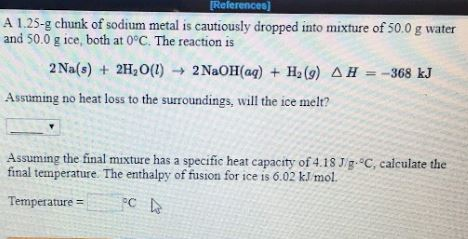# A. 1.25-g chunk of sodium metal is cautiously dropped into mixture of 50.0 g water and 50.0 g ice, both at 0 degree C. The reaction is 2Na(s) + 2H2O(l) → 2NaOH(aq) + H2(g) H = -368 kJ Assuming no heat loss to surroundings, will ice melt? Assuming the final mixture has a specific heat capacity of 4.18 J/g degree C, calculate the final temperature. The enthalpy of fusion for ice is 6.02 kJ/mol Temperature = degree C